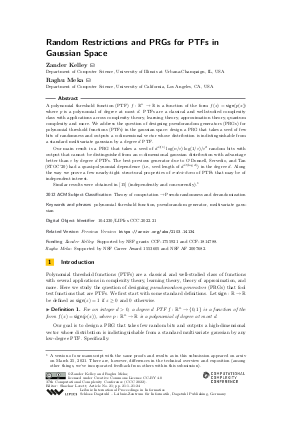Document# Random Restrictions and PRGs for PTFs in Gaussian Space

### Authors Zander Kelley, Raghu Meka## File

LIPIcs.CCC.2022.21.pdf
• Filesize: 0.74 MB
• 24 pages

## Cite As

Zander Kelley and Raghu Meka. Random Restrictions and PRGs for PTFs in Gaussian Space. In 37th Computational Complexity Conference (CCC 2022). Leibniz International Proceedings in Informatics (LIPIcs), Volume 234, pp. 21:1-21:24, Schloss Dagstuhl - Leibniz-Zentrum für Informatik (2022)
https://doi.org/10.4230/LIPIcs.CCC.2022.21

## Abstract

A polynomial threshold function (PTF) f: ℝⁿ → ℝ is a function of the form f(x) = sign(p(x)) where p is a polynomial of degree at most d. PTFs are a classical and well-studied complexity class with applications across complexity theory, learning theory, approximation theory, quantum complexity and more. We address the question of designing pseudorandom generators (PRGs) for polynomial threshold functions (PTFs) in the gaussian space: design a PRG that takes a seed of few bits of randomness and outputs a n-dimensional vector whose distribution is indistinguishable from a standard multivariate gaussian by a degree d PTF. Our main result is a PRG that takes a seed of d^O(1) log(n/ε) log(1/ε)/ε² random bits with output that cannot be distinguished from an n-dimensional gaussian distribution with advantage better than ε by degree d PTFs. The best previous generator due to O'Donnell, Servedio, and Tan (STOC'20) had a quasi-polynomial dependence (i.e., seed length of d^O(log d)) in the degree d. Along the way we prove a few nearly-tight structural properties of restrictions of PTFs that may be of independent interest. Similar results were obtained in [Ryan O'Donnell et al., 2021] (independently and concurrently).

## Subject Classification

##### ACM Subject Classification
• Theory of computation → Pseudorandomness and derandomization
##### Keywords
• polynomial threshold function
• pseudorandom generator
• multivariate gaussian

## Metrics

• Access Statistics
• Total Accesses (updated on a weekly basis)
0

## References

1. Ido Ben-Eliezer, Shachar Lovett, and Ariel Yadin. Polynomial threshold functions: Structure, approximation and pseudorandomness. CoRR, abs/0911.3473, 2009. URL: http://arxiv.org/abs/0911.3473.
2. Ilias Diakonikolas, Daniel M Kane, and Jelani Nelson. Bounded independence fools degree-2 threshold functions. In 2010 IEEE 51st Annual Symposium on Foundations of Computer Science, pages 11-20. IEEE, 2010.3. Ilias Diakonikolas, Prasad Raghavendra, Rocco A. Servedio, and Li-Yang Tan. Average sensitivity and noise sensitivity of polynomial threshold functions. SIAM J. Comput., 43(1):231-253, 2014. URL: https://doi.org/10.1137/110855223.
4. Prahladh Harsha, Adam Klivans, and Raghu Meka. Bounding the sensitivity of polynomial threshold functions. Theory of Computing, 10(1):1-26, 2014. URL: https://doi.org/10.4086/toc.2014.v010a001.
5. Valentine Kabanets, Daniel M Kane, and Zhenjian Lu. A polynomial restriction lemma with applications. In Proceedings of the 49th Annual ACM SIGACT Symposium on Theory of Computing, pages 615-628, 2017.6. Daniel M Kane. k-independent gaussians fool polynomial threshold functions. In 2011 IEEE 26th Annual Conference on Computational Complexity, pages 252-261. IEEE Computer Society, 2011.7. Daniel M Kane. A small PRG for polynomial threshold functions of gaussians. In 2011 IEEE 52nd Annual Symposium on Foundations of Computer Science, pages 257-266. IEEE, 2011.8. Daniel M Kane. A structure theorem for poorly anticoncentrated gaussian chaoses and applications to the study of polynomial threshold functions. In 2012 IEEE 53rd Annual Symposium on Foundations of Computer Science, pages 91-100. IEEE, 2012.9. Daniel M Kane. The correct exponent for the Gotsman-Linial conjecture. In 2013 IEEE Conference on Computational Complexity, pages 56-64. IEEE, 2013.10. Daniel M Kane. A pseudorandom generator for polynomial threshold functions of gaussian with subpolynomial seed length. In 2014 IEEE 29th Conference on Computational Complexity (CCC), pages 217-228. IEEE, 2014.11. Daniel M Kane. A polylogarithmic PRG for degree 2 threshold functions in the gaussian setting. In Proceedings of the 30th Conference on Computational Complexity, pages 567-581, 2015.12. Raghu Meka and David Zuckerman. Pseudorandom generators for polynomial threshold functions. SIAM Journal on Computing, 42(3):1275-1301, 2013.13. Ryan O'Donnell. Analysis of boolean functions. Cambridge University Press, 2014.14. Ryan O'Donnell, Rocco A Servedio, and Li-Yang Tan. Fooling gaussian PTFs via local hyperconcentration. In Proceedings of the 52nd Annual ACM SIGACT Symposium on Theory of Computing, pages 1170-1183, 2020.15. Ryan O'Donnell, Rocco A. Servedio, Li-Yang Tan, and Daniel Kane. Fooling gaussian PTFs via local hyperconcentration, 2021. arXiv:2103.07809. URL: http://arxiv.org/abs/2103.07809.
X

Feedback for Dagstuhl Publishing